PSABM5WX - Practice---Acc7.5 Lesson 12 On Both of the Lines (8.EE.C.8)
Part A)

Diego has $11 and begins saving$5 each week toward buying a new phone. At the same time that Diego begins saving, Lin has $60 and begins spending$2 per week on supplies for her art class. Is there a week when they have the same amount of money?

Select one:
Part B)

After how many weeks?

Type your answer below as a number (example: 5, 3.1, 4 1/2, or 3/2):
Part C)

How much do they have at that time?

Type your answer below as a number (example: 5, 3.1, 4 1/2, or 3/2):
Part A)

Use a graph to find x and y values that make bothandtrue.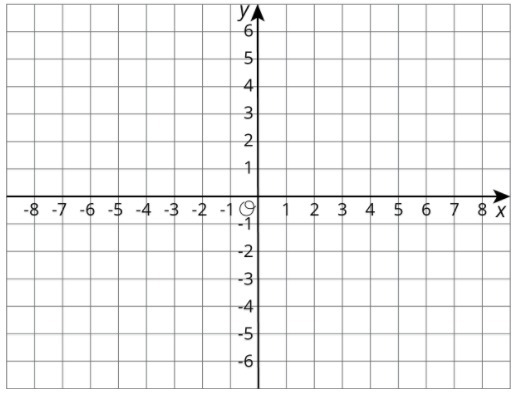Draw your graph on paper, take a picture, and upload it using the image upload icon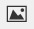If you do not have the ability to upload an image of your work type "Graph is on paper."

Part B)

What are the x and y values that make both equations true?

(x,y)

with no spaces

The point where the graphs of two equations intersect has y-coordinate 2. One equation is. Find the other equation if its graph has a slope of 1.

Complete the equation below

y = _______________

Part A)

A farm has chickens and cows. All the cows have 4 legs and all the chickens have 2 legs. All together, there are 82 cow and chicken legs on the farm. Complete the table to show some possible combinations of chickens and cows to get 82 total legs.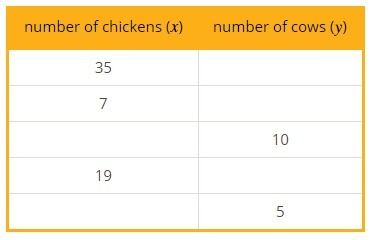Create and fill in the table using the table button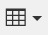Part B)

Here is a graph that shows possible combinations of chickens and cows that add up to 30 animals: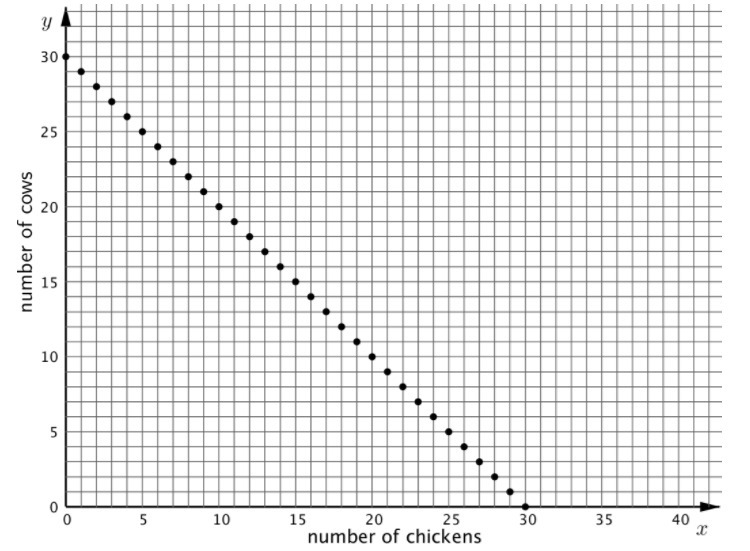If the farm has 30 chickens and cows, and there are 82 chicken and cow legs all together, then how many chickens and how many cows could the farm have?

Chickens:

Type your answer below as a number (example: 5, 3.1, 4 1/2, or 3/2):
Part C)

Cows:

Type your answer below as a number (example: 5, 3.1, 4 1/2, or 3/2):
Part A)

Match the lines m and n to the statements they represent: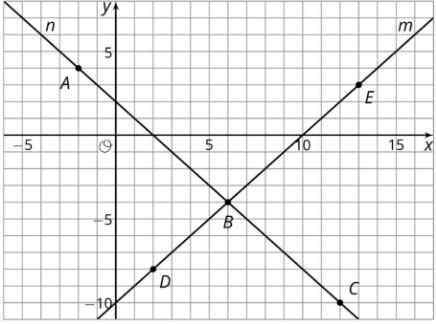Statement: A set of points where the coordinates of each point have a sum of 2.

Select one:
Part B)

Match the lines m and n to the statements they represent:

Statement: A set of points where the y-coordinate of each point is 10 less than its x-coordinate.

Select one:
Part C)

Match the labeled points on the graph to statements about their coordinates:

Statement: Two numbers with a sum of 2

Select all that apply:
Part D)

Match the labeled points on the graph to statements about their coordinates:

Statement: Two numbers where the y-coordinate is 10 less than the x-coordinate

Select all that apply:
Part E)

Match the labeled points on the graph to statements about their coordinates:

Statement: Two numbers with a sum of 2 and where the y-coordinate is 10 less than the x-coordinate

Select all that apply:
Part A)

Here is an equation: 4− 4__. What could you write in the blank so the equation would be true for:

No values of x

Part B)

Here is an equation: 4− 4__. What could you write in the blank so the equation would be true for:

All values of x